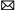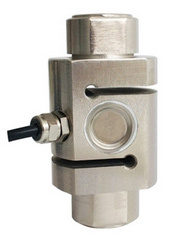inquiry@ato.com.cn
+1 800-585-1519 (Toll-free)

Home » Load Cell » 100kg/500kg/20 ton Load Cell Elastic Element Construction Analysis

# 100kg/500kg/20 ton Load Cell Elastic Element Construction Analysis

The normal stress annular elastic elements are mostly the integrated circular structure with the upper and lower double-screw bolts or the integrated annular structure. Under compression load, the elastic annulus and the assembled structure made up of additional pressure head and base wad are usually adopted. It is a tension and compression load cell with capacity from 100kg to 20 ton.If there are special requirements on electronic hook scale, the rectangular annular elements can also be adopted. In order to improve the processing manufacturability, the internal annulus of the rectangular ring can be connected by three connecting holes, including one large central hole and two side holes. The upper and lower loading part of the rectangular elastic elements can be double-screw bolts or pulling ears connected by hinge pins. The integrated annular structure with the upper and lower double-screw bolts and the integrated plate annular structure with the upper and lower screw holes.
1. Structural and mechanical analysis of the integrated annular structure with the upper and lower double-screw bolts
The integrated annular structure with the upper and lower double-screw bolts makes full use of the bending stress of the annulus, which is characterized by a large strain utilization coefficient and high output sensitivity; good linearity and small lag. The upper and lower double-screw bolts are capable of pulling load and compression load double-direction loading. The resistance strain gauge is pasted in the internal hole of the annulus to gain good protection. It is particularly suitable to the pulling load detection of electronic lifting hook and so on. The defect is mainly reflected as the huge difficulty of mechanical processing, heat processing and prevention sealing.
The annulus mainly makes use of the bending stress of the B-B cross-section and the loading axis cross-section. Since the corner of B-B cross-section in loading deformation is zero, B-B cross-section is usually regarded as a fixed terminal. Through theoretical calculation, the strain and stress of Point B and the sensitivity of annular elastic elements can be obtained.
The moment of Point B is MB＝0.182PR
The axial force of Point B is:
N=P/2
The bending stress generated by MB can be written as below:
σ1=MB(h/2)/J=6MB/bh2
The uniformly distributed normal stress generated by N can be written as below:
σ2=N/A=N/(bh)=P/(2bh)
According to the superposition principle of force, the stress and strain of Point B can be written as below, respectively:
σB12=6MB/bh2+P/2bh
εB=6MB/bh2E+P/2bhE
Sensitivity of elastic elements can be written as below:
S=(6MBK/bh2E+PK/2bhE)*103mV/V
2. Structural and mechanical analysis of the integrated plate annular structure with the upper and lower screw hole
In order to overcome the defects of elastic elements with an integrated annular structure with the upper and lower double-screw bolts, the integrated plate annular structure with the upper and lower screw holes is adopted. A striking characteristic is that the partial circular structure with the external ring is turned into the simple rectangular structure with a geometric shape. By processing the rims of four small holes and opening holes, the annular effect is achieved for the plate ring. In this way, the manufacturability of mechanical processing and heat processing can be improved. Meanwhile, performance fluctuations caused by the dimension, form and local tolerance can be reduced. In this way, accuracy and stability of elastic elements are superior to the integrated annular structure.
The plate ring is usually simplified into the annular ring for computing. Since the error of computing results is huge, the method which obtains the modification coefficient based on the actually-measured value is adopted.
Assume that the average radius of the plate ring is R0, the inner bore radius is R1, the outer ring radius is R2 and the width is h.
R0=(R1+R2)/2
According to R0 /h, the ratio of the average radius to the width, the modification coefficient, G, the sensitivity calculation formula can be written as below:
S=G*(3KR0P/2bh2E)*103
Take P＝10000kg plate-ring elastic element of load cell for example. The structural dimension is the inner circle radius, R1＝25mm, the ex-radius is R2＝50mm, and the horizontal cross-sectional wall thickness is b＝45mm.
Hence,
R0=(R1+R2)/2=(25+50)/2=37.5mm
h=R2-R1=50-25=25mm
R0/h=37.5/25=1.5
Based on the modification coefficient curve, it can be obtained that G=0.9. Then, the sensitivity of the 10,000kg (10 ton) plate-ring elastic element can be written as below:
S=0.9*[(3*2.1*37.5*1000)/(2*4*252*2.1*104)]*103=1.8mV/V

I have an Opinion:

Plz Calculate (4 - 1) =
(Your comment will show after approved.)

You may also like: